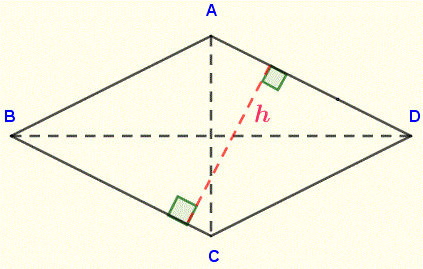### Popular Pages# Rhombus Calculator and Solver

Online calculators to calculate the area, perimeter, diagonals and angles of a rhombus.
The notations to be used for the inputs to the calculator are the one adopted in the figure of the rhombus ABCD shown below. Angle A is opposite diagonal BD and angle B is opposite diagonal AC. h is the distance between two opposite sides of the rhombus; it is called the height.There are four calculators and solvers that may be used depending on which parameters you have and what you need to know.

## 1 - Rhombus Calculator given the side and one angle

Enter the side and one angle (in degrees) as positive real numbers and press "Solve Rhombus". The outputs are: the area, perimeter, the two diagonals, angle B and the height.

 side = 10 , Angle A = 30 Decimal Places = 4 Area = Perimeter = Diagonal BD = Diagonal AC = Angle B = height h =

## 2 - Rhombus Calculator given the two diagonals

Enter the two diagonals as positive real numbers and press "Solve Rhombus". The outputs are: the area, perimeter, side, angles A and B and the height.

 Diagonal BD = 10 , Diagonal AC = 30 Decimal Places = 4 Side = Area = Perimeter = Angle A = Angle B = height h =

## 3 - Rhombus Calculator given the area and the perimeter

Enter the
area and perimeter as positive real numbers and press "Solve Rhombus". The outputs are: the side; the two angles, the two diagonals and the height.

 Area = 10 , Perimeter = 30 Decimal Places = 4 Side = Diagonal BD = Diagonal AC = Angle A = Angle B = height h =

## 4 - Rhombus Calculator given the height and one diagonal

Enter the
height and one diagonal as positive real numbers and press "Solve Rhombus". The outputs are: the side, the area, the perimeter, the two angles and the second diagonals .

 height = 10 , Diagonal BD = 30 Decimal Places = 4 Side = Diagonal AC = Perimeter = Area = Angle A = Angle B =